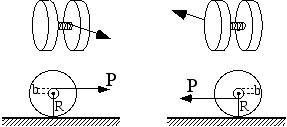# Rolling SpindleHow would I find the acceleration of the above spindles (both left and right instances)? The spindles are composed of two disks with a cylindrical solid axle. The left spindle is unwinding the string as it rolls and the right spindle is winding the string as it rolls. Static friction is present and the spindle rolls without slipping. Any help would be greatly appreciated.

I know that the applied force P minus the force of friction equals the mass times acceleration. I can't seem to figure out how to find the force of friction, with which it would be relatively easy to solve the problem.

Doc Al
Mentor
Originally posted by cpuwildman
I know that the applied force P minus the force of friction equals the mass times acceleration. I can't seem to figure out how to find the force of friction, with which it would be relatively easy to solve the problem.
Two equivalent ways to go:

(1) Consider all the forces on the spindles and apply Newton's 2nd law both for translation (of center of mass) and rotation (about center of mass).

(2) Treat the spindle as instantaneously in pure rotation about the point of contact with the surface, taking torques about that point.

I tried the torque method and summed all of the torques and came up with the force of friction for the left spindle as (MRa + 1/2mba) where M is the mass of the large disc, m is the mass of the axle, R is the radius of the large discs, b is the radius of the axle, and a is the acceleration. For some reason I still don't seem to get the correct answer. After getting the above result, I subtracted that from the applied force which equals the total mass times acceleration. I solved for the acceleration and got a wrong answer. I can't submit my homework any more, but I would still like to figure out how to do this as I have a test coming up next week. I really appreciate your help.

ShawnD
For the picture on the left, the sum of the moments looks like this, not following sign convention (just let everything be positive here).

$$\sum M = (P)(R + b)$$

The picture on the right is like this

$$\sum M = (P)(R - b)$$

The moment and angular acceleration formula is like this

$$\sum M = I \alpha$$

$$\alpha = \frac{\sum M}{I}$$

If you want real acceleration, just multiply by the radius of the wheel (just R)

$$a = \frac{r \sum M}{I}$$

I too have a test on this stuff. It's on friday

Last edited:
Doc Al
Mentor
Originally posted by cpuwildman
I tried the torque method and summed all of the torques and came up with the force of friction for the left spindle as (MRa + 1/2mba) where M is the mass of the large disc, m is the mass of the axle, R is the radius of the large discs, b is the radius of the axle, and a is the acceleration. For some reason I still don't seem to get the correct answer. ...
I have no idea how you arrived at that answer. You need to apply Newton's 2nd Law for (1) translation and (2) rotation. Here's what I get for the diagram on the left.

(1) $$P - F = Ma$$

(2) $$Pb + FR = I \alpha$$

Where F is the friction force; M is the total mass; I is the rotational inertia about the axle; a is acceleration; &alpha; is angular acceleration. Solve these two equations together and you'll get your answer. (Also realize that a = &alpha;R.)

You have to model the spindle in order to calculate the rotational inertia. You didn't give any information, so I don't know what you can assume. (For example, can you ignore the axle and treat the spindle as two cylinders? I would.)

Give it another shot.

If you wanted to use the other method that I spoke of--where you treat the spindle as in pure rotation--it would look like this: (This is what ShawnD was doing.)

$$P(R + b) = (I + MR^2) \alpha$$

Note that since you are taking torques about the contact point, you need the rotational inertia about that point; for that I used the parallel axis theorem.

Edit: added note on second method.

Last edited: